# 2018上C语言程序设计（高级）作业- 第3次作业

### 作业要求一 ：

PTA作业的提交列表：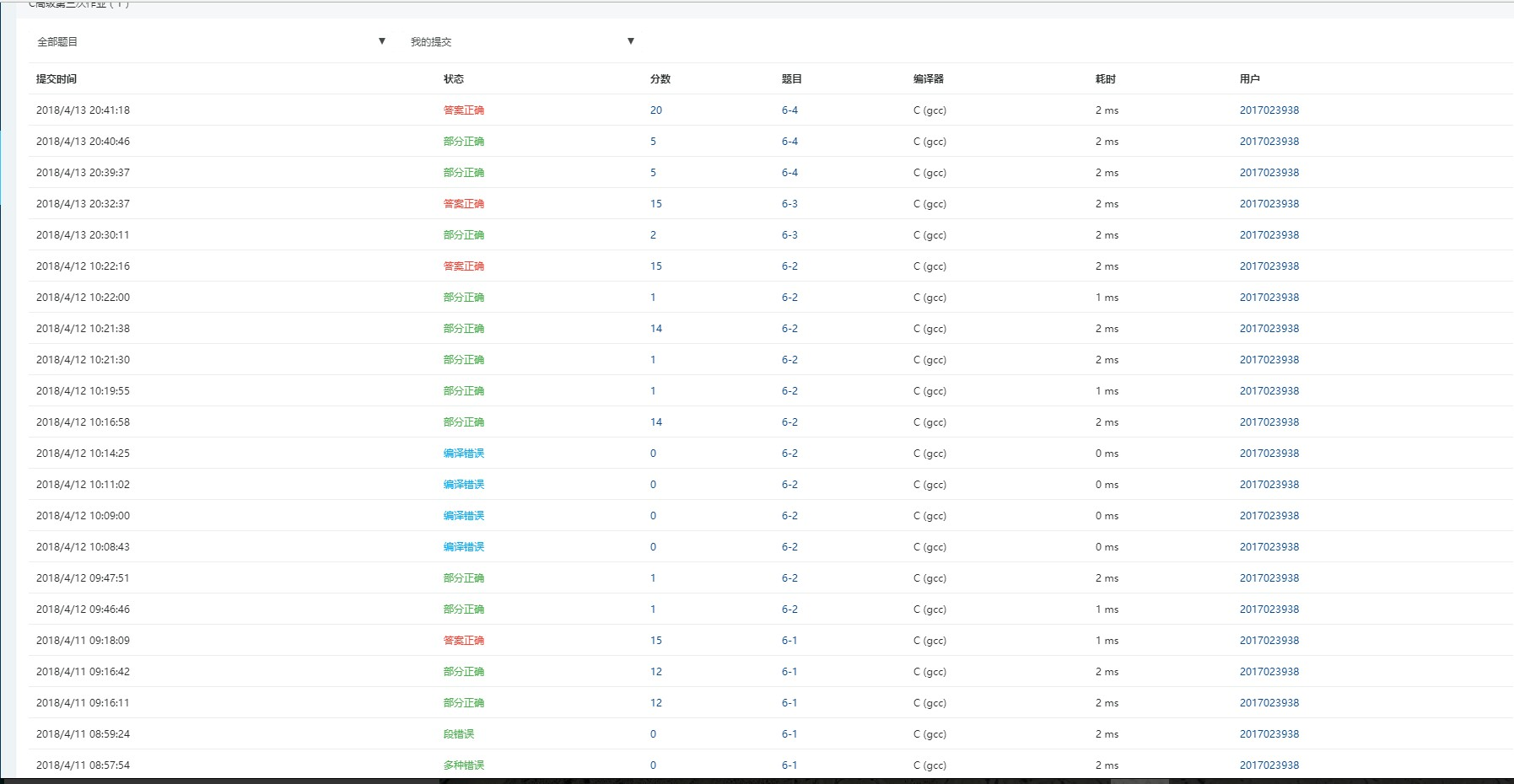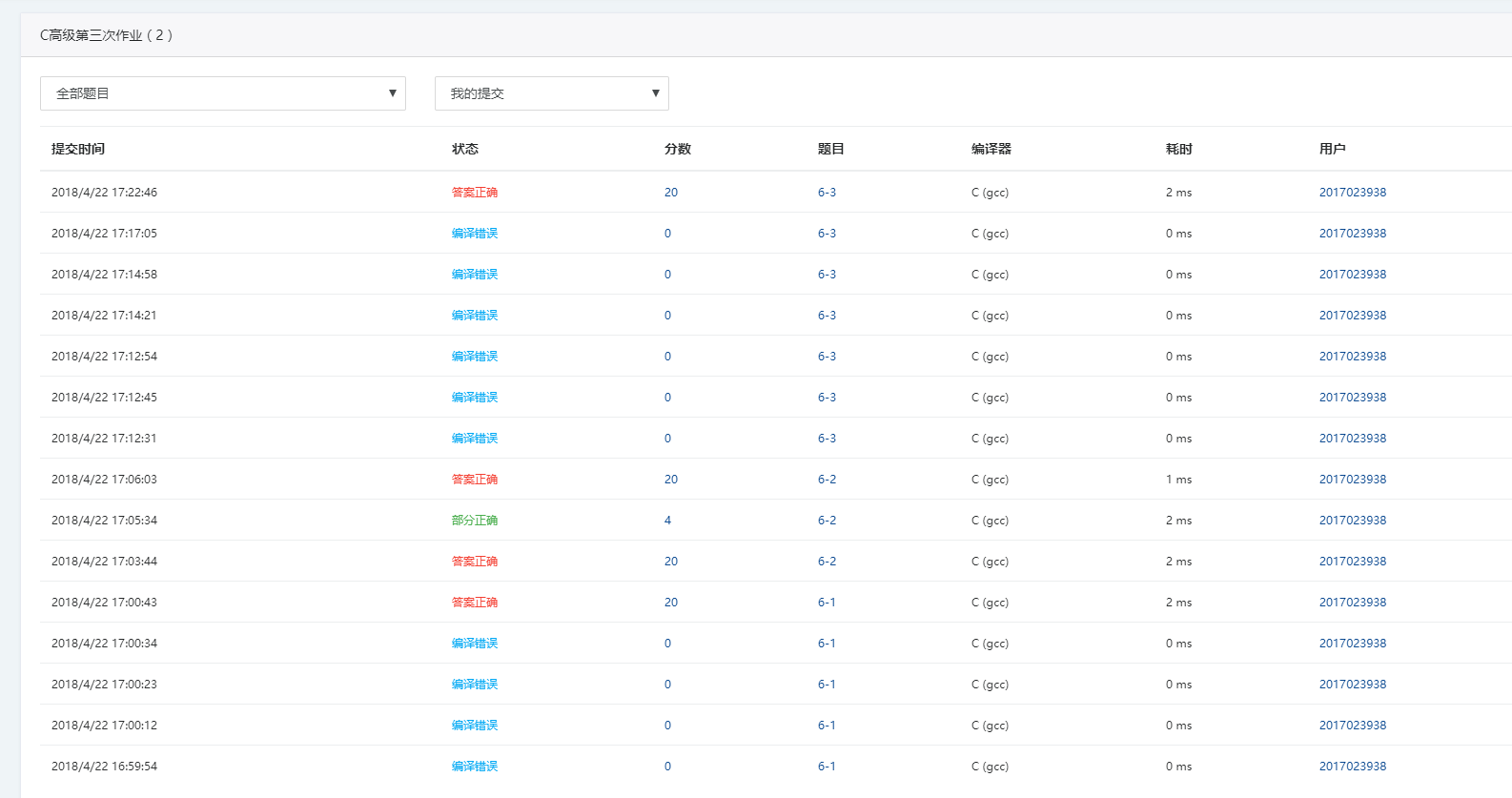### 作业要求二：

#### 6-1 输出月份英文名

1.设计思路

（1）主要描述算法：

（2）流程图：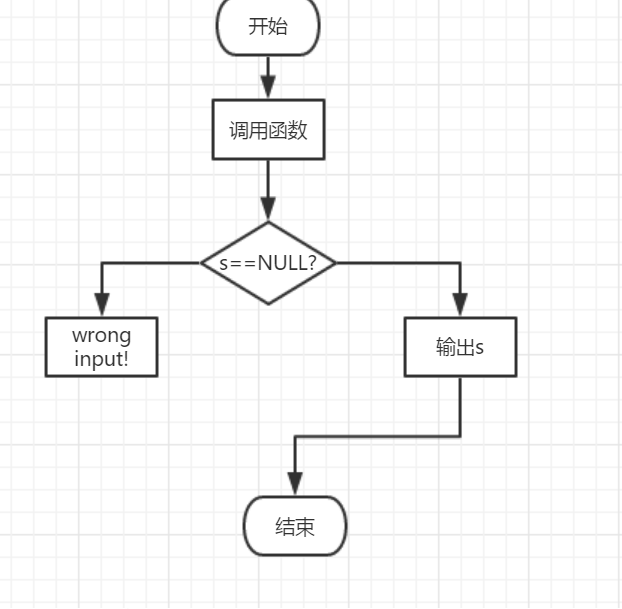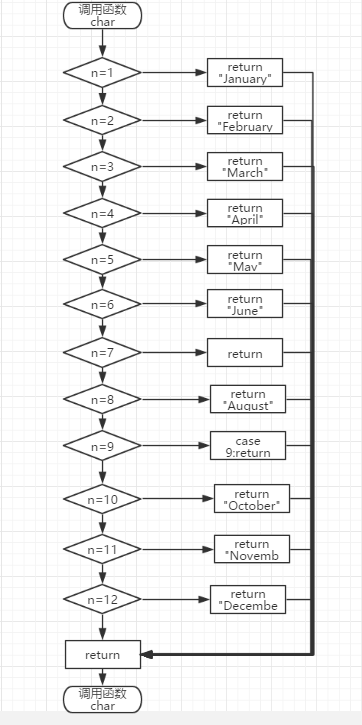2.实验代码：

char *getmonth( int n )
{
switch(n)
{
case 1:return "January";
case 2:return "February";
case 3:return "March";
case 4:return "April";
case 5:return "May";
case 6:return "June";
case 7:return "July";
case 8:return "August";
case 9:return "September";
case 10:return "October";
case 11:return "November";
case 12:return "December";
default:return NULL;
}
} 

3.本题调试过程碰到问题及解决办法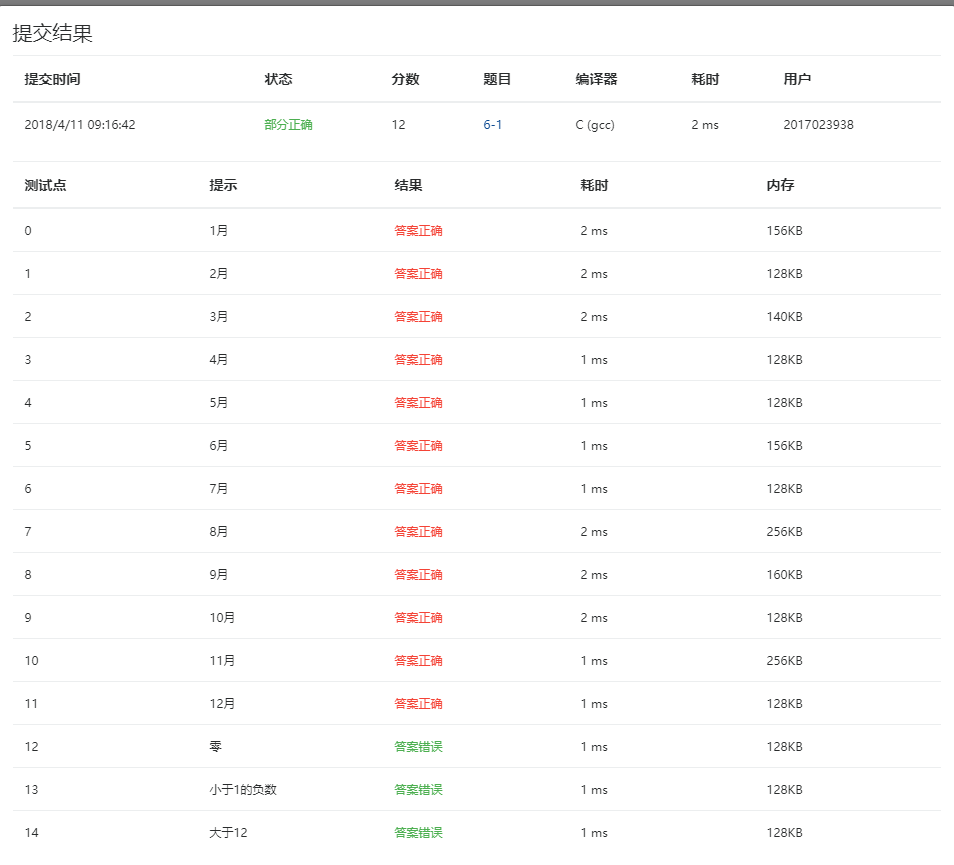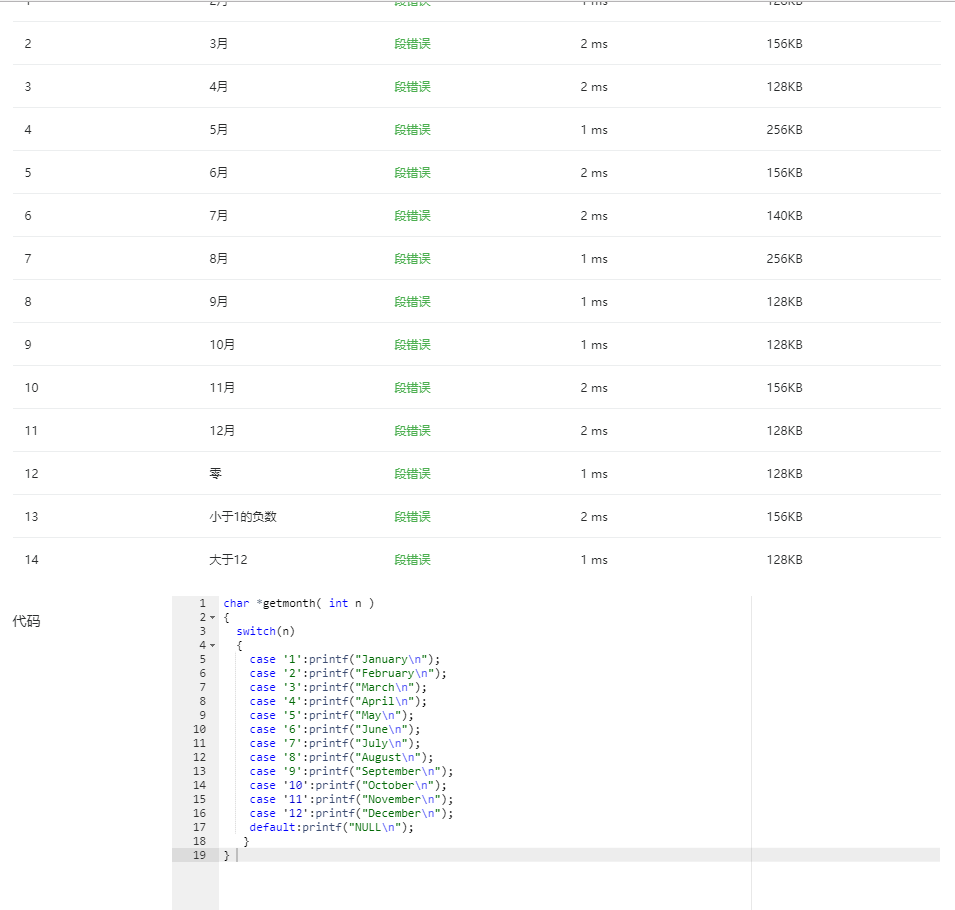遇到的问题2：case后面的数字用单引号括了起来。

#### 6-2 查找星期

1.设计思路

2.实验代码：

int getindex( char *s )
{
int i,m=-1;
char *week={"Sunday","Monday","Tuesday","Wednesday","Thursday","Friday","Saturday"};
for(i=0;i<7;i++)
{
if (strcmp (s, week[i]) == 0)
{
m=i;
break;
}
}
return m;
} 

3.本题调试过程碰到问题及解决办法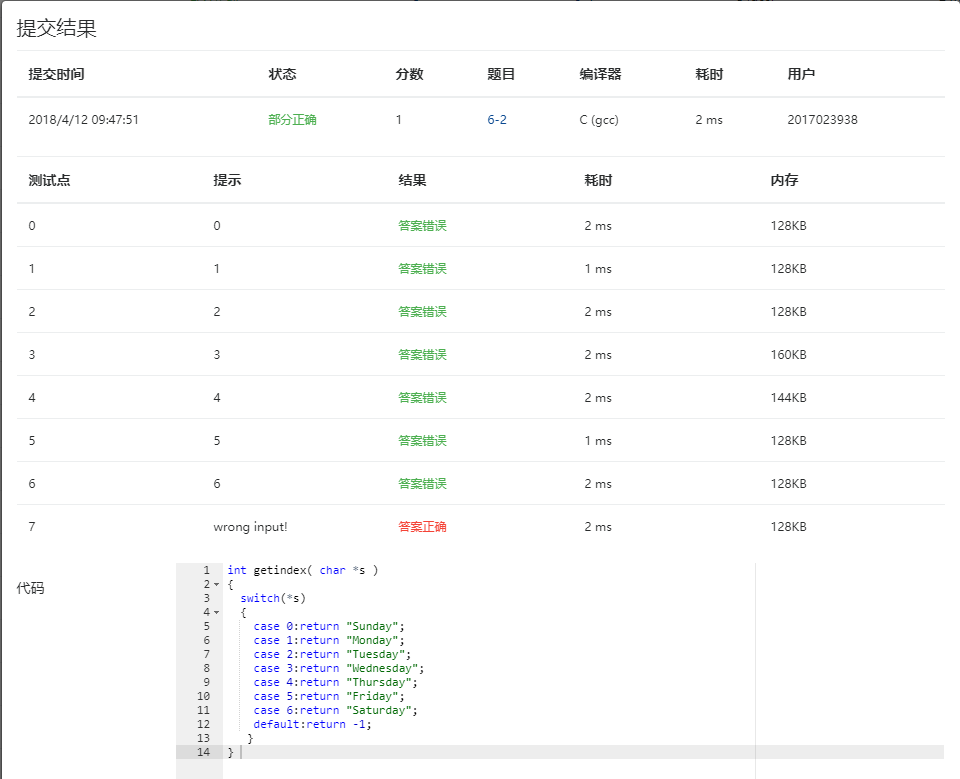#### 6-3 计算最长的字符串长度

1.设计思路

2.实验代码：

int max_len( char *s[], int n )
{
int i,j,m={0};
int count=0;
for(i=0;i<n;i++)
{
for(j=0;*(*(s+i)+j)!='\0';j++)
{
}
m[i]=j;
}
for(i=0;i<n;i++)
{
if(m[i]>count)
{
count=m[i];
}
}
return count;
} 

3.本题调试过程碰到问题及解决办法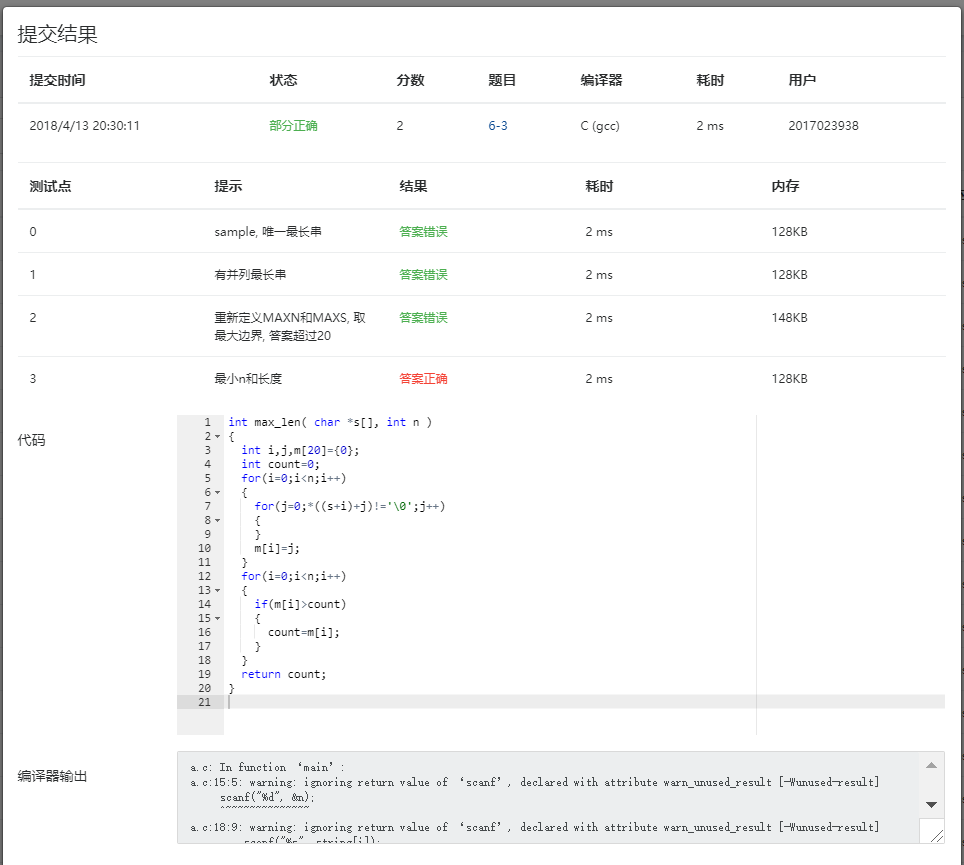#### 6-4 指定位置输出字符串

1.设计思路

2.实验代码：

char *match( char *s, char ch1, char ch2 ){
int i,j;
for(i=0;*(s+i)!='\0';i++)
{
if(*(s+i)==ch1)
{
char *a=  &s[i];
for(j=i;(*(s+j)!=ch2)&&(*(s+j)!='\0');j++)
{
printf("%c",*(s+j));
}
if(*(s+j)!='\0')
printf("%c",*(s+j));
printf("\n");
return a;
}
}
printf("\n");
return s+i;
}

3.本题调试过程碰到问题及解决办法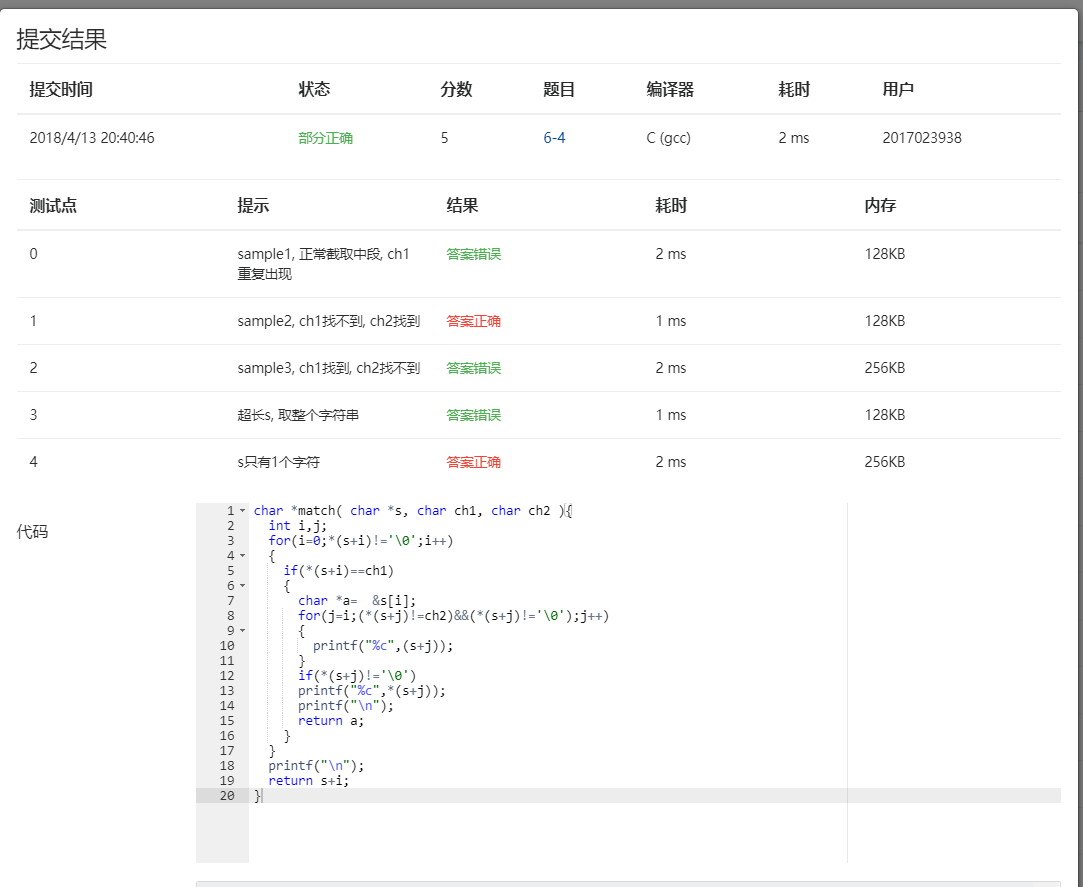#### 6-1 奇数值结点链表

1.设计思路

（2）流程图：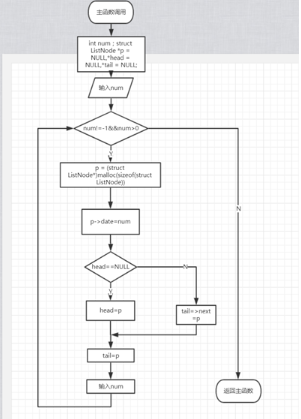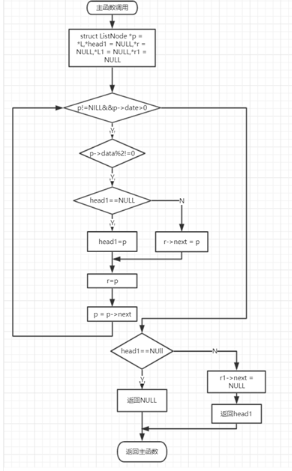2.实验代码：

struct ListNode *readlist() {
int number;
struct ListNode *p = NULL,*head = NULL,*tail = NULL;
scanf("%d",&number);
while(number!=-1 && number>0 )
{
p = (struct ListNode*)malloc(sizeof(struct ListNode));
p->data = number;
{
} else
{
tail->next = p;
}
tail = p;
scanf("%d",&number);
}
{
return NULL;
}
tail->next = NULL;
}
struct ListNode *getodd( struct ListNode **L )
{
struct ListNode *p = *L,*head1 = NULL,*r = NULL,*L1 = NULL,*r1 = NULL;
while(p!=NULL && p->data>0)
{
if(p->data%2!=0)
{
{
} else
{
r->next = p;
}
r = p;
} else
{
if(L1 ==NULL)
{
L1 = p;
} else
{
r1->next = p;
}
r1 = p;
}
p = p->next;
}
{
return NULL;
} else
{
r->next = NULL;
}
if(L1==NULL)
{
*L = NULL;
} else {
r1->next = NULL;
*L = L1;
}
}

3.本题调试过程碰到问题及解决办法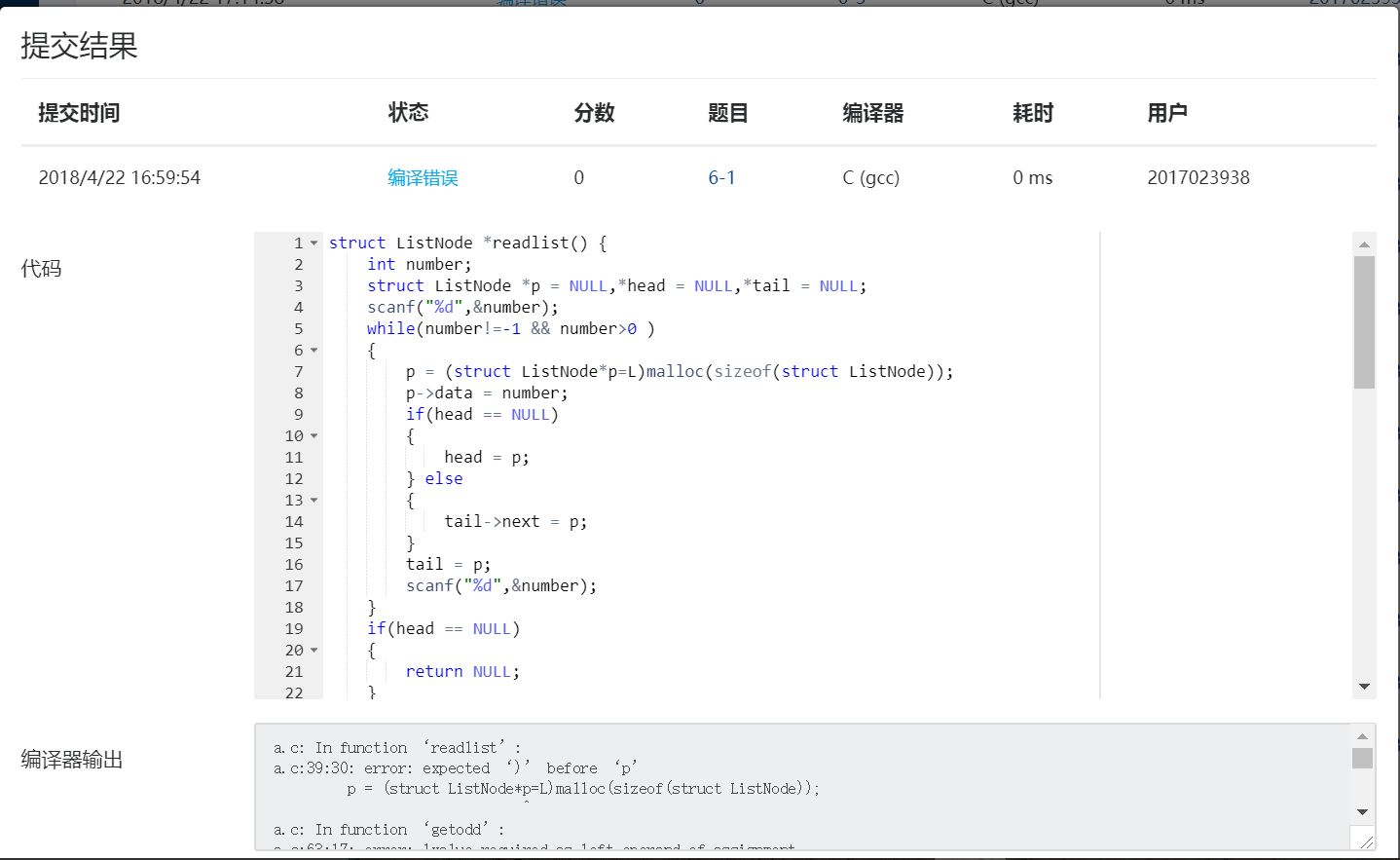#### 6-2 学生成绩链表处理

1.设计思路

2.实验代码：

struct stud_node *createlist()
{
int num,score;
char name;
p = (struct stud_node*)malloc(sizeof(struct stud_node));
scanf("%d",&num);

while (num != 0)
{
p = (struct stud_node *)malloc (sizeof (struct stud_node));
scanf ("%s %d", p->name, &p->score);
p->num=num;

else
tail->next = p;
tail = p;
scanf ("%d", &num);
}
}
struct stud_node *deletelist( struct stud_node *head, int min_score )
{
while(L!=NULL)
{
if(L->score>=min_score)
{
{
} else
{
tail1->next = L;
}
tail1 = L;
}
L=L->next;
}
{
return NULL;
} else
{
tail1->next =NULL;
}
} 

3.本题调试过程碰到问题及解决办法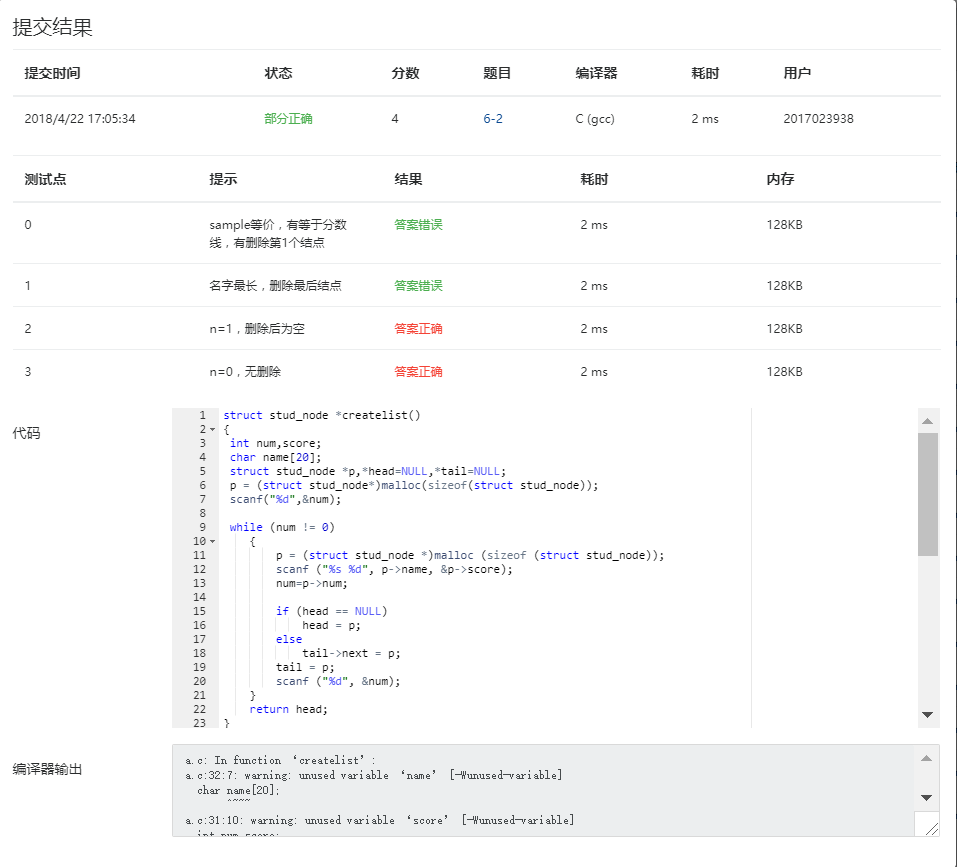#### 6-3 链表拼接

1.设计思路

2.实验代码：

struct ListNode *mergelists(struct ListNode *list1, struct ListNode *list2)
{
int num = 0;
int temp;
struct ListNode  *p = list1;
while(p != NULL)
{
temp[num] = p->data;
num++;
p = p->next;
}
p = list2;
while(p != NULL)
{
temp[num] = p->data;
num++;
p = p->next;
}
int i,j;
for(i = 0; i < num; i++)
for(j = i + 1; j < num; j++)
{
if(temp[i] > temp[j])
{
int t;
t = temp[i];
temp[i] = temp[j];
temp[j] = t;
}
}
struct ListNode  *newlist = NULL;
struct ListNode  *endlist = NULL;
struct ListNode  *q;
for(i = 0; i < num; i++)
{
q = (struct ListNode  *)malloc(sizeof(struct ListNode));
q->data = temp[i];
if(newlist == NULL)
{
newlist = q;
newlist->next = NULL;
}
if(endlist != NULL)
{
endlist->next = q;
}
endlist = q;
endlist->next = NULL;
}
return newlist;
}



3.本题调试过程碰到问题及解决办法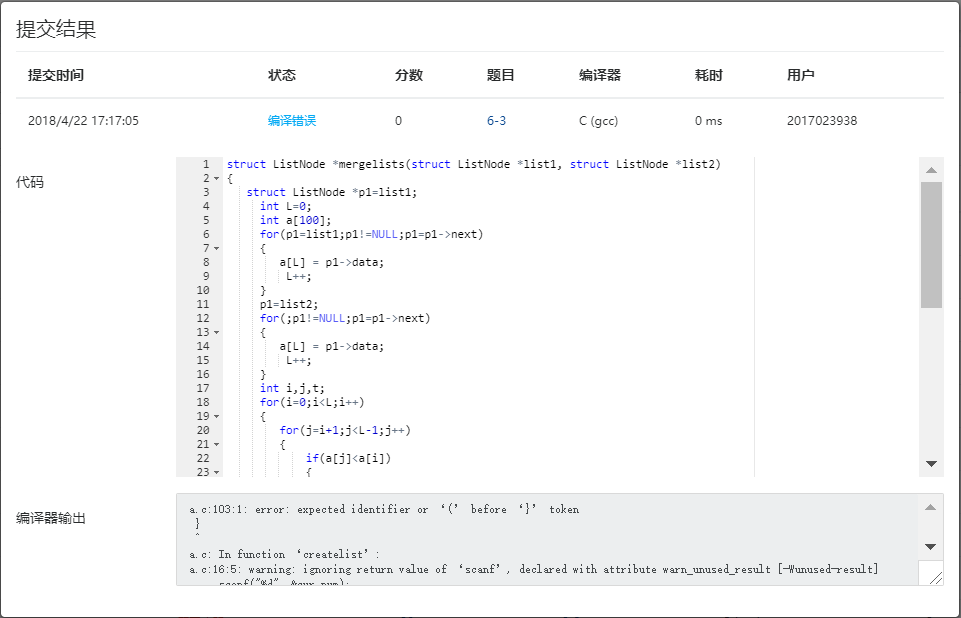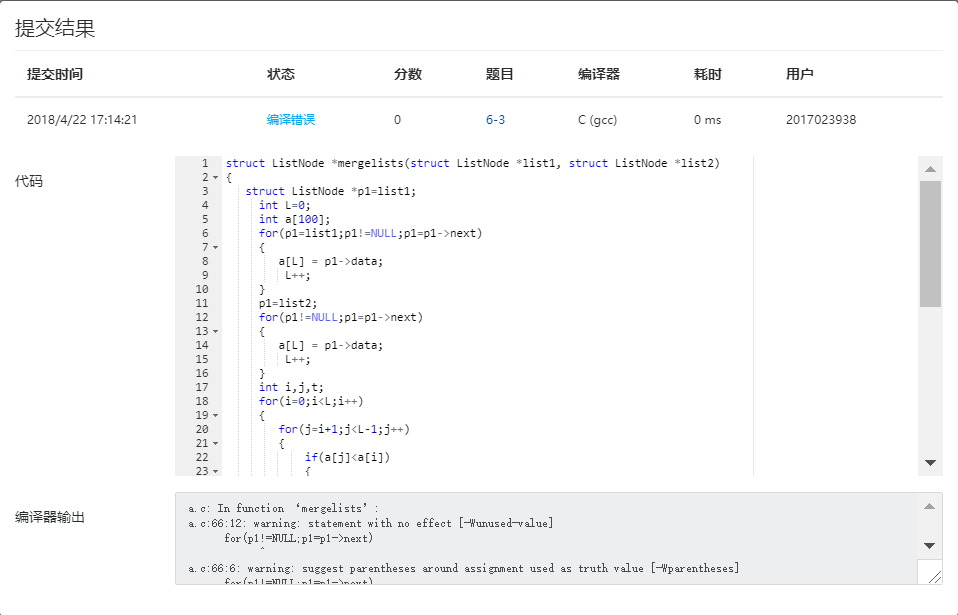#### 要求三、学习总结和进度：

1、总结两周里所学的知识点，回答下列问题？（用自己的话表达出你的理解，网上复制粘贴没有分数）（5分）

（1）如何理解指针数组，它与指针、数组有何关系？为何可以用二级指针对指针数组进行操作？

指针数组是指向内存地址的数组，使用指针数组更节省空间；二维数组定义存在数据区（可更改）

（2）将C高级第三次PTA作业（1）任何一个题目改为使用二级指针对指针数组进行操作。

目前对这一部分的内容掌握得不是很好。

（3）用指针数组处理多个字符串有何优势？可以直接输入多个字符串给未初始化的指针数组吗？为什么？

使用指针数组处理多个字符串更节省空间，因为每个字符串是单独存放的；不可以，未初始化，会出现一些错误；点评同学：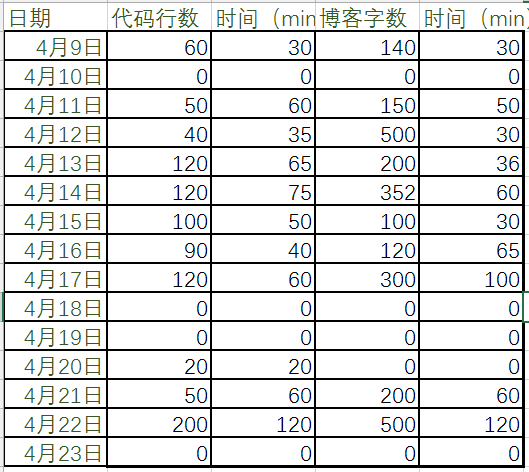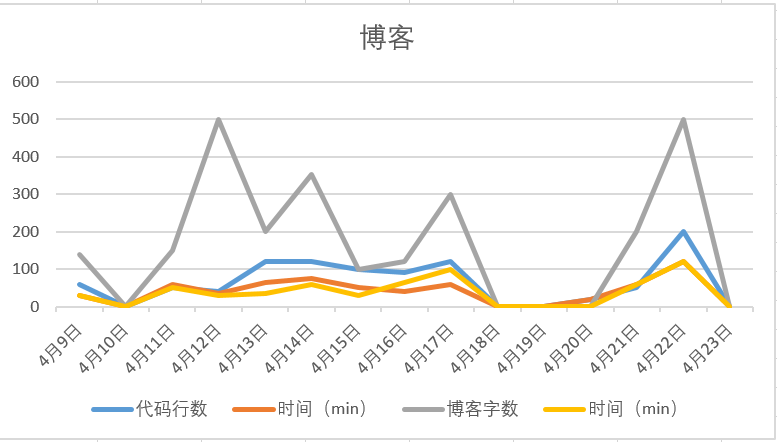posted @ 2018-04-15 13:46  Suzy.F  阅读(1681)  评论(10编辑  收藏  举报